# Nuclear, Particle, and Atomic Physics

With the recent publication of PHYSICS IS... there are now three Ask the Physicist books! Click on the book images below for information on the content of the books and for information on ordering.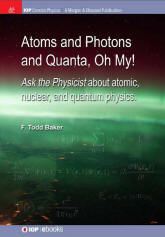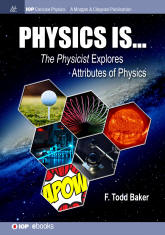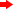QUESTION:
I recently watched with great interest a PBS program which described in layman's terms how Uranium 238 transforms into the different chained elements, to include U235. It also explained the basics of the chain reaction caused by splitting U235 using E=MC^2 as the basis for energy release. This is where I was a little unclear. The split was described as one U325 nucleus splitting into two separate nuclei with some individual particles released (can't remember if they are protons or neutrons) Those particles then collide with other U235 atoms in proximity triggering subsequent splits and particle releases as part of a chain reaction. My question is that the mass doesn't appear to be transforming into energy (E=MC^2). Rather it appears that it is simply splitting and being cast off, so what causes the energy release? This assumes that the number of particles in the remaining two nuclei + the particles independently released still equal 235. There was a general reference in the program to how the Strong Force reduces the size of the resultant smaller nuclei, but it didn't say if matter within each was converted to energy or if the number of particles are additionally reduced through such a conversion. Thanks for any clarification you can provide.ANSWER:
Suppose that you weigh one 235U and one neutron. Now, when you add the neutron to the 235U it fissions and, after all is said and done you have two lighter atoms and a few neutrons; if you weigh all these byproducts, you will find that approximately 0.1% of the original weight is missing. Where did it go? Most of it went into kinetic energy of the byproducts, that is they are all moving faster. Kinetic energy of atoms is essentially what thermal energy is
—the reactor (or bomb) has gotten hotter. For a bomb it all gets enormously hotter resulting in the explosion. For a reactor, the rate of fissioning is controlled and the heat is extracted to drive turbines to create electricity. More detail can be found in an earlier answer.FOLLOWUP QUESTION:
I'm assuming (correct me if I'm wrong) that such motion was some percent of the speed of light which would account for the transformation of about 0.1% of its matter to energy.ANSWER:
First things first—there was an error in my original answer, now corrected throughout: the amount of mass converted to energy is about 0.1%; nuclear fusion is about 1%. No, the motion of the atoms is nowhere near the speed of light. It is simply classical
½mv2 type of kinetic energy. The "transformation" is simply that—mass energy transformed into kinetic energy. To understand, see a recent answer which explains why a bound system has less mass than if it is pulled apart and the mass measured. It turns out that heavy nuclei like uranium are less tightly bound than nuclei with roughly half their mass; therefore when they split the products are less massive. That is why fission works as an energy source.QUESTION:
I don't know if you know about the device scientist called the torus experiment. I heard about it at UGA thirty years ago. It was to make a round tube with wire around all of it. Then special particles went through the tube, and the device put out a lot of energy. They tried to make one 5 miles in diameter, but they kept having problems with centrifugal force. The machine would make as much electricity as a nuclear power plant, or more. What I thought of is real simple, but maybe it was overlooked or something. My idea is to make the shape like a track around a football field, and make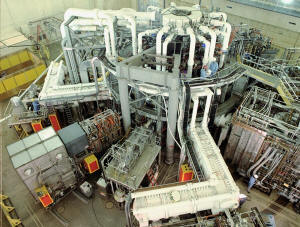the straight parts a lot longer. So the particles would have time to realign in the center and all start going the same speed. My teacher said it is like a contained nuclear explosion. It would put out a lot more electricity than it takes to run it.ANSWER:
What you are describing sounds like a tokamak, an experimental fusion reactor. Nuclear fusion is the energy which powers stars. Roughly, the idea is that if you get hydrogen atoms hot enough (hundreds of millions of degrees) they will have enough energy to fuse together to make helium, and that releases a great deal of energy. The problem turned out to be much more challenging than anticipated and there still is not a practicable fusion reactor which will give you more energy out than you use to run it. The main problem was the difficulty of keeping the hot plasma confined long enough to have a sufficient number of fusion reactions. The current research is centered on constructing a tokamak by an international collaboration known as ITER; the lab is in France. There is an old cynical joke among physicists: "Fusion is the energy fo the future and always will be!"QUESTION:
I am really confused over the spin of neutrino, my teacher says it is always spin 1/2, however with my calculation from beta decay it can be 3/2 also? Please clarify why it can't be spin 3/2?ANSWER:
I have no idea how you can calculate the spin of a particle from the decay. Spin is the quantum number of the intrinsic angular momentum of a particle, one of the properties of the particle itself, and cannot be anything other than what it is,
½ in the case of a neutrino (or electron, or proton, or neutron). A spin-½ particle might also have an orbital angular momentum quantum number L and its total angular momentum quantum number J=L±½ could be different from its spin. Maybe that is how you are coming up with 3/2. For example, if L=1 for the neutrino, J=½ or 3/2. (By  the way, if you are just learning about angular momentum, the actual angular momentum of an object with angular momentum quantum number N is (h/2π)√(N(N+1)) where h is Planck's constant.)QUESTION:
Since the rest mass of the constituent quarks only makes up about 2% of the total mass of the proton (or neutron), would you please explain in layman's terms what it is in QCD that forces all protons (or neutrons) to have the exact same mass. Does the answer to this question imply that time is quantized?ANSWER:
One of the things which the uncertainty principle says is that you cannot know precisely both the energy and the time of a quantum system, ΔEΔt
h/2π where h/2π=6.6x10-16 eV·s is the rationalized Planck's constant. In order to measure the energy of a system, you must spend some time; the more time you have to examine the system, the more accurately you can determine its mass. But, if the system is unstable, that is it has some lifetime before it decays to a different state, you will get a different answer every time you try to measure its mass (which is, equivalently, its energy). A proton is a stable particle and therefore, since it lives forever its mass has zero uncertainty and is why all protons have identically the same mass. However, you are wrong that all neutrons have the same mass because the neutron beta-decays to a proton+electron+neutrino with a half life of about 880 s. So, the uncertainty in the neutron's rest mass energy is about ΔEh/(2πΔt)=7.5x10-19 eV=7.5x10-28 GeV. The rest mass energy of a neutron is about 1 GeV, so the uncertainty is about 7.5x10-26 %! This is small but not zero. These results have nothing to do with either the masses of quarks or time quantization.QUESTION:
Is it correct to say that nuclear fusion violates the law of conservation of mass since a portion of the mass is converted into photons?ANSWER:
Well, you could say that if there were such a law as conservation of mass. Since 1905 when Albert Einstein showed us that mass is just another form of energy, the only valid such law is conservation of energy. Even in chemistry where conservation of mass appears to be correct, the ultimate source of energy is mass being changed into kinetic energy of the chemical reaction products (heat); chemistry is such an inefficient source of energy that the mass changes are miniscule.QUESTION:
Can you tell me why muons are extremely unstable (lasting only fractions of a second) while electrons and neutrinos are pretty much stable? I just don't get why muons are so unstable since they're just leptons like electrons and neutrinos just more massive than the other two.ANSWER:
If you look at the decay of the muon, you will see that the mass of the products, an electron and two neutrinos, is much less than the mass of the muon. This means that the decay is energetically possible, energy is released by the decay so the decay products have kinetic energy afterwards. In nature, almost always when a process is energetically possible it will occur. Only in cases where a decay would be prohibited by some selection rule will decay not occur. For example, a proton cannot decay into three electrons because charge conservation would be violated, even though it is energetically possible.QUESTION:
I'm writing a research paper for my college english class and the topic is Thorium Reactors. My question is "Are thorium based reactors such as LFTR fusion or fission and based?" I was just wondering because the it seems from what I've learnt that the reactors use the thorium to produce a reaction that makes another element such as uranium 233 which I assume is fusion because I'm sure they're using the energy put off from that initial reaction to power something. But after the uranium 233 is used and to produce energy as efficiently as possible I would think that you would implement a system that would immediately and directly use said produced uranium into some form a fission reactor.ANSWER:
Fusion always involves light nuclei and there is no such thing as a fusion reactor, only ideas for them. So a thorium reactor must be a fission reactor. It would be inaccurate, though, to call thorium the fuel because thorium is not fissile. If a thermal neutron is absorbed by a fissile nucleus, it will fission and result in more neutrons leading to more fissions and the reaction can be self-sustaining. Thorium, which is 100% 232Th, absorbs a neutron to become 233Th which
β-decays to 233Pa (half life 22 minutes)which β-decays to 233U (half life 27 days). The 233U is fissile and is nearly stable (α-decay half life 160,000 years). Thorium is said to be fertile, absorption of a neutron results in production of a fissile fuel. So a thorium reactor is a breeder reactor, a reactor whose purpose is create fuel. At the startup one needs a starter fissile fuel  as well as the thorium to provide neutrons to create the 233U. As the 233U builds up, it becomes the fuel.QUESTION:
Why is it necessary to have a minimal temperature of 150 million degrees Kelvin for nuclear fusion on earth if the sun does nuclear fusion at a temperature of 15 milloin degrees?ANSWER:
There is more than one reason that I can think of. The mass of the sun is 2x1030 kg, quite a bit bigger than the mass of fuel in a fusion reactor. Therefore the rate of fusion in the sun can be low but the energy output would still be huge. Increasing the temperature would increase the rate. The density of the sun's core is about 150 g/cm3, 150 times more dense than water. In a fusion reactor, the practical densities are many orders of magnitude below this. Again, the rate of fusion would be dependent on the density of the fuel.QUESTION:
I've just found out that a proton isn't made up of two up and one down quark but also contains zillions of other up and down quarks along with their anti matter equivalents. Here's a link to an article from the L.H.C people at Cern that shows this. I'm amazed and confused because I thought that matter and anti matter particles would annihilate each other. Why don't they? And, if they do, how is the 'zillions of other quarks' balance maintained?ANSWER:
The crux of what is going on here is that a vacuum is not really a vacuum as we generally think of it
—nothing. Particle-antiparticle pairs are continuously popping into existence and then annihilating back to nothing after a short time. This is called virtual pair production. Also, if a particular particle experiences a particular force, the messenger of that force (gluons for the strong interaction, photons for the electromagnetic interaction) are continuously being emitted and reabsorbed. All this is called vacuum polarization. So, I could give you a similar description of the hydrogen atom: the hydrogen atom is not really a proton and an electron, it is a proton and zillions of electrons and positrons and photons. If you really want to understand the hydrogen atom in detail, you need to take the effects of vacuum polarization into account (see the Lamb shift, for example). The CERN explanation should have included zillions of positrons and electrons and photons inside the nucleus also. Just as a hydrogen atom is pretty well described as a proton and an electron as a first approximation, a proton is pretty well described as three quarks as a first approximation.QUESTION:
Why the whole matter of radioactive sample does not disintigrate at once or Why it always take half life to disintegrate half of initial value?ANSWER:
Because decay is a statistical process. Whenever you have a large number N of anything they will, at some time t, have a time rate of change R=dN/dt. If R<0, N is getting smaller (as in radioactivity) and if R>0, N is getting larger (like bacteria growth). For a great many cases in nature, it turns out that the rate is proportional to the number, R
N. Radioactivity turns out to behave that way: if you measure R for 2x1020 radioactive nuclei it will be twice as big than when you measure R for 1020 nuclei. You can then solve for what R is for a given situation: dN/dt=-λN where λ is the proportionality constant, called the decay constant; the minus sign is put there so that λ will be a positive number since the rate is negative. If you know differential equations, you will find that N=N0e-λt where N0 is the number when t=0. The decay constant is related to the half life τ½=ln(2)/λ. However, this all depends on there being a large number to start. In the extreme case, if N0=1, they would all decay at once! You just could not predict when. If N0=2, they might both go at once or else one might go before the other but not necessarily at τ½.QUESTION:
Electrons orbit around the nucleus in varying degrees of proximity to the nucleus. Do electrons farther from the nucleus orbit at a different rate of speed than those closer to the nucleus?ANSWER:
Yes, if you use a Bohr model for the electrons. However, the notion of electrons running around in well-defined orbits is naïve and incorrect.FOLLOWUP QUESTION:
Would it be correct to apply Kepler's Laws of Motion to the revolution of electrons about the nucleus in the Electron Cloud Model?ANSWER:
The electrostatic force is a 1/r2 force just like the gravitational force, so if the atom were a classical system Kepler's laws could be used for an atom. In fact, the Bohr-Sommerfeld model, the first extension of the Bohr's circular orbit model, essentially does this by including elliptical orbits and appropriate quantization. Of course, the atom is not a classical system and although such models can be instructive, they are not strictly accurate. What you refer to as the "electron cloud model" would be the proper solutions to the Schrodinger equation, not having well-defined orbits.QUESTION:
I really don't understand why the detonation of a nuclear weapon does not cause fusion in the gasses in our atmosphere. (Which as I understand was a concern, albeit not a big one in the Manhattan Project.) The detonation of such a device certainly provides more than enough heat and pressure; or so I would think. A little internet searching reveals that thermonuclear devices utilize fission devices to both heat and compress hydrogen to achieve the reaction. So why does one need to add a hydrogen to the fission device to achieve fusion, rather than just rely on the gasses in our atmosphere? Or is it simply that a lot more energy is required to get something like Oxygen or Nitrogen to undergo fusion? All I could come up with thinking of a possible solution was that the shockwave of compressed air these weapons create is made up of gasses that moved away from the source of heat before they could reach that critical temperature, am I on the mark or way off?ANSWER:
There are many reasons why your ideas will not work.

• Fusion requires bringing two charged nuclei close together and the energy required to bring them close enough together to fuse is proportional to the product of their charges. Nitrogen would require 49 and oxygen 64  times the energy to fuse compared with hydrogen.

• The thermonuclear device is designed to compress and confine the fusion fuel using the fission device energy to do this. If you just had a fission bomb, the surrounding air molecules would be blown apart from each other rather than compressed.

• There is also efficiency to consider. In hydrogen fusion, about 1% of the mass of the fuel is converted to energy but for oxygen or nitrogen, only about 0.1% is.

• Finally, you have to ask whether the desired reaction will go at all and what its probability (cross section) is. My guess is that there would be a very low probability for 14N+14N—>28Si to occur.QUESTION:
How much energy is required to move the electron sufficiently far away from the proton such that it does not experience the proton's electric field. I am kind of confused by this question. The effect of electric field will never end as far as we take the electron from the proton. And if we use the relation V=E.L then if E=0, then L will be infinity?ANSWER:
Technically, yes the field will be exactly zero only at L= ∞.
But, that is only if your proton and your electron are the only things in the universe! You can calculate the ionization energy, the energy necessary to move an electron from the ground state of hydrogen to infinity; it is 13.6 eV=2.18x10-18 J. You can also calculate the energy necessary to move it to any other distance. For example, the energy to move it to about 0.5 μ=5x10-7 m (which is about 100 times the radius of the hydrogen atom) is 13.599 eV, essentially the same as to infinity. So you can see that most of the energy is supplied in close to the proton because that is where the field (and therefore the force) is strongest.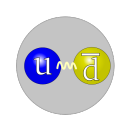QUESTION:
So I've been trying to figure this for a while, if a positively charged and a negatively charged quark orbit each other or actually come in contact, also could they form a functional "atom" and be able to have electrons interact with them?ANSWER:
Two-quark particles are called mesons (one quark plus one antiquark). If you had a positively- and negatively-charged quark you would have an uncharged meson and so it would not bind an electron and you could not have a meson+electron atom. However, if you had a positively charged pion, it could bind an electron. For example, a positive pion π+ is composed of an up quark (charge +2e/3) and a down antiquark (charge +e/3) and would have a charge +e and be able to form an "atom" which you might call pionium.ADDED NOTE:
Whoops! Pionium is an "atom" composed of one π+ and one π-. I cannot find any reference to this pion+electron system, so I guess you can call it whatever you like.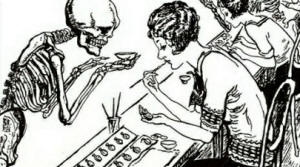QUESTION:
I read from my history book that when nukes were first created we didn't know that the radiation their explosions produce is harmful to one's health, even to one's life maybe. So my question is, how come neither physicists or medical personnel ever figured out that radiation can be dangerous until we had first victims of radiation?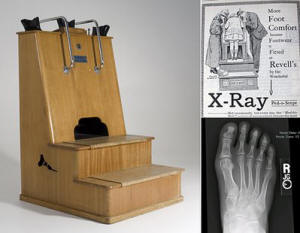ANSWER: